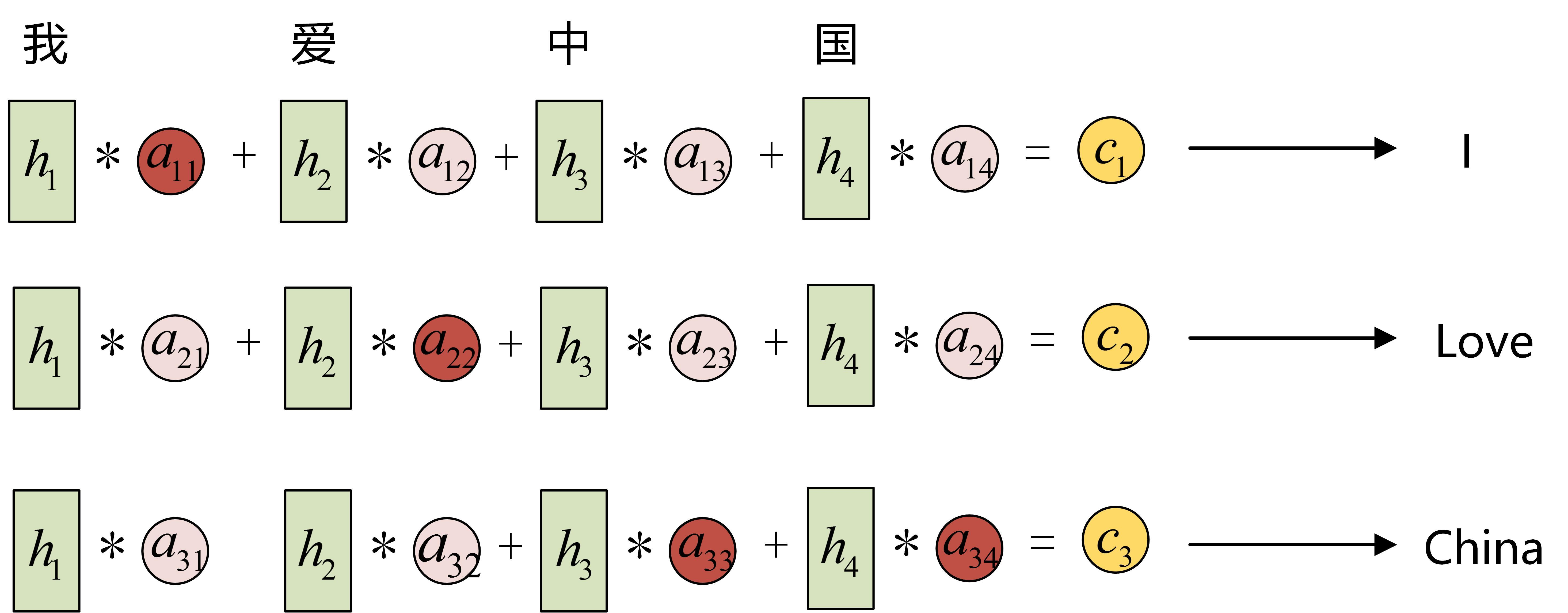# Seq2seq模型及注意力机制

ryluo 2020-06-14 01:29:22

Seq2Seq模型的结构及序列模型注意力的理解

### Seq2Seq模型

#### 编码器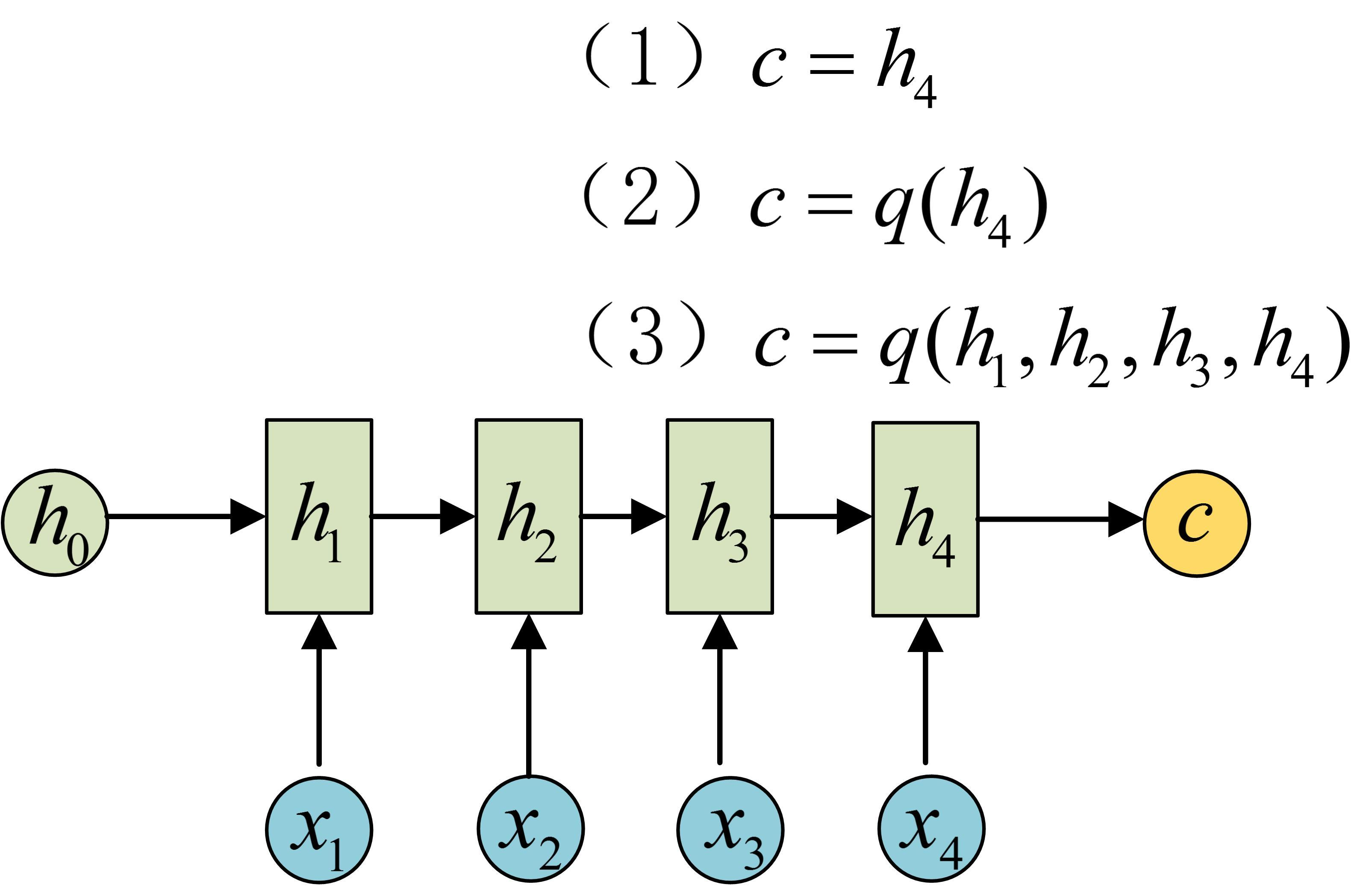#### 解码器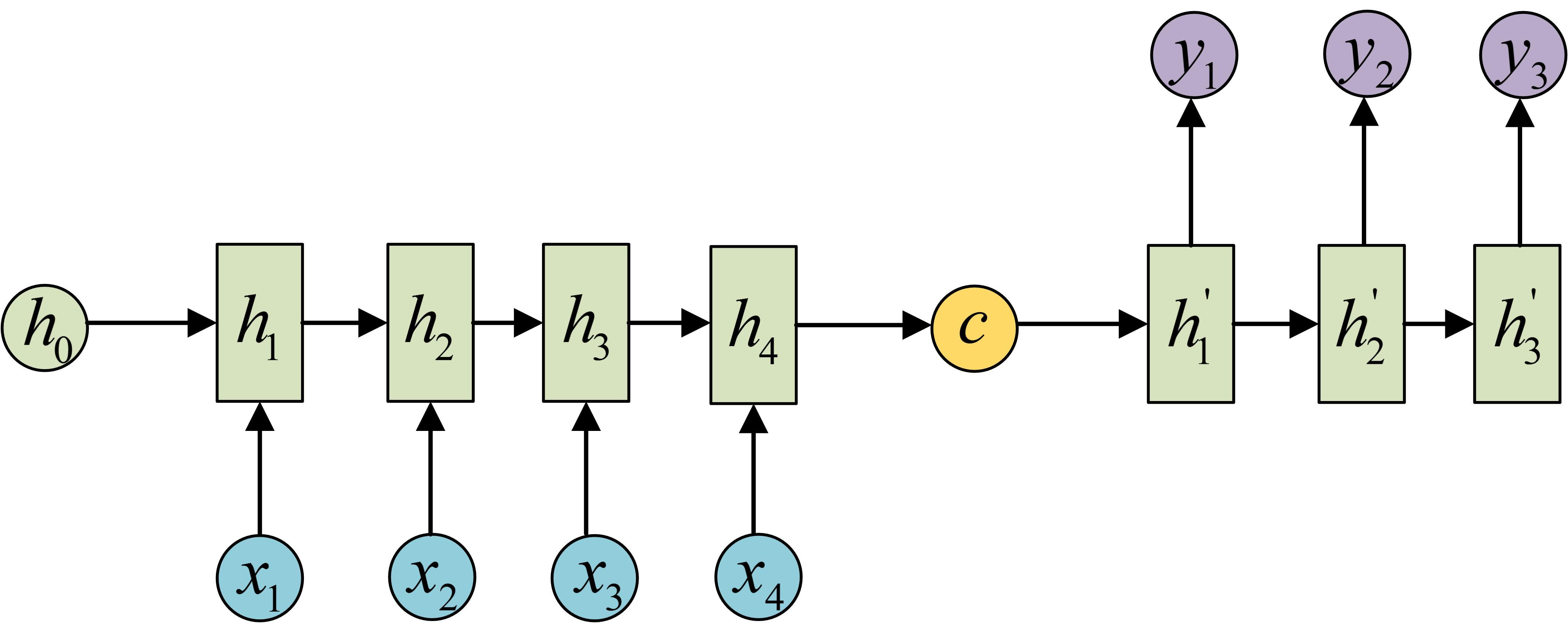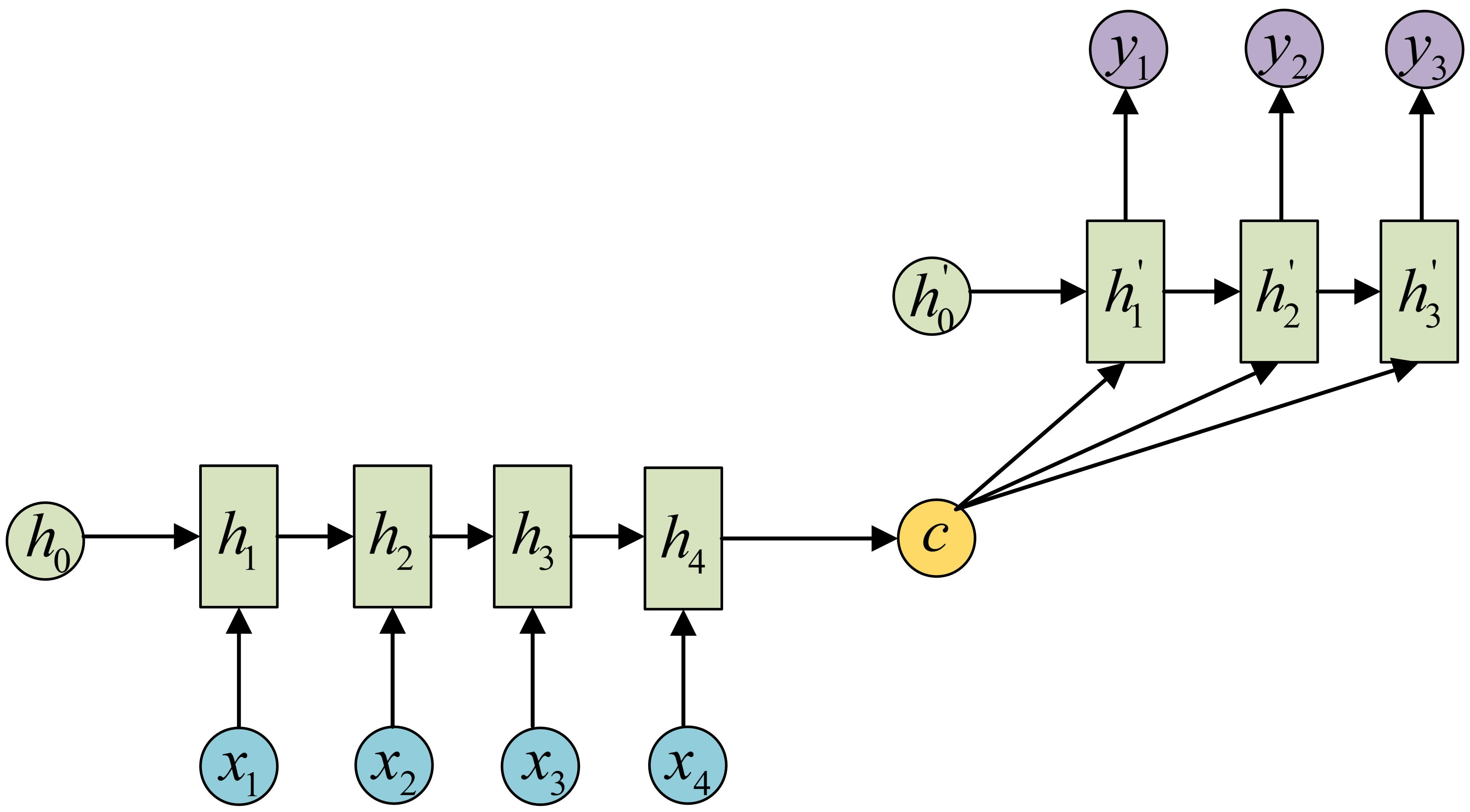### Attention机制的Seq2Seq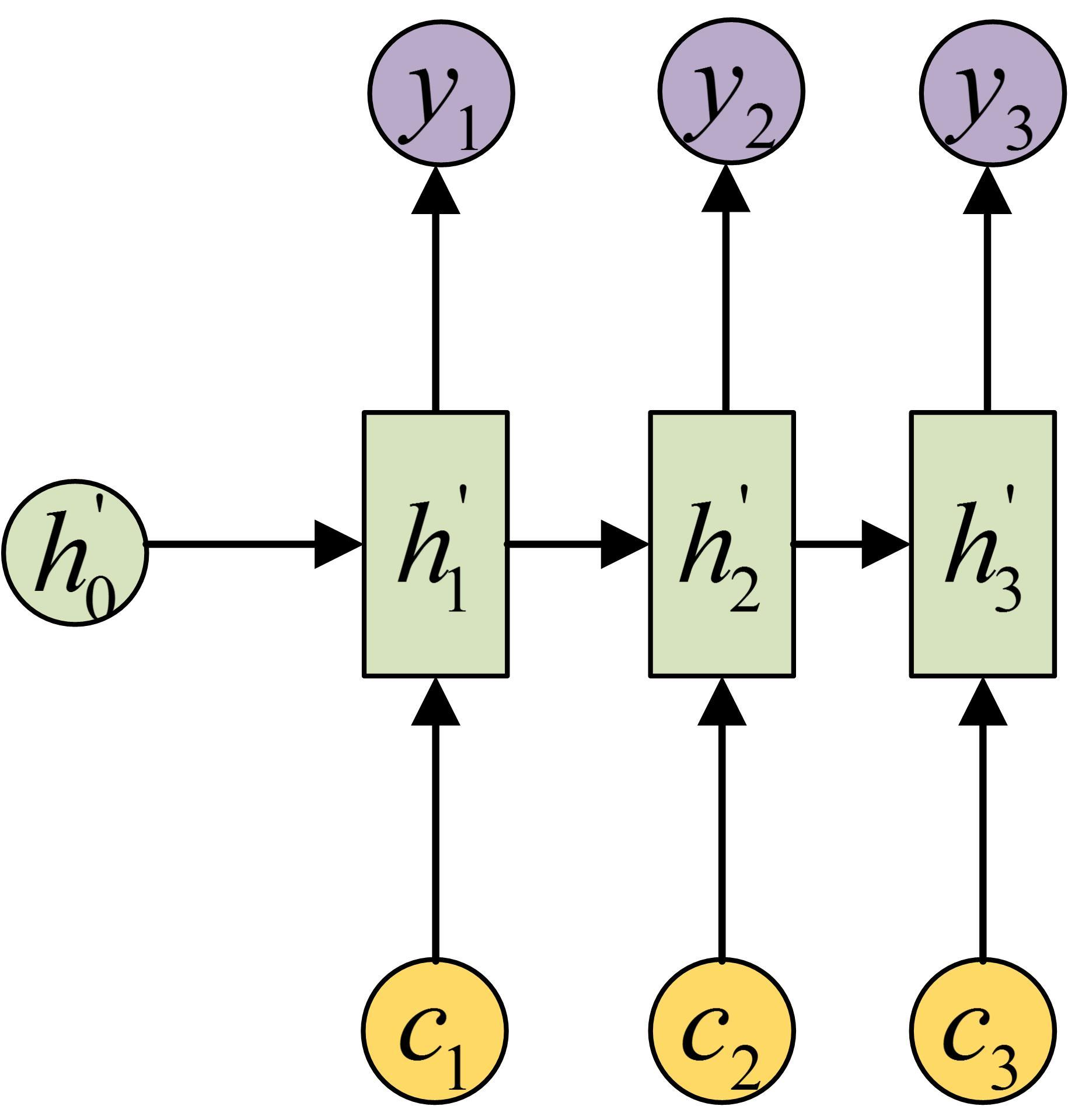Attention机制生成的上下文向量可以自动的去选取与当前时间步输出最有用的信息，用有限的上下文向量的容量去表示当前时间步对输入信息最关注的那部分信息，最简单的做法就是对编码器输出的所有时间步的隐藏状态进行一个加权平均，不同的权值所对应的隐含状态就是对不同时间步的输入信息关的注程度，如下图就很清楚的说明了具体是怎么操作的(下图来自《动手学深度学习》第447页)。图中的$a$表示是编码器不同时间步对应的权值，而其权值又决定于编码器该时间步的隐藏状态以及解码器上一个时间步的隐藏状态(这里直接这么说比较绕，很容易看不明白，建议去看课本上的解释)。下面给出一个简单的解释：设解码器当前隐藏状态为$s_{t’}$

$y_{t’-1}:$解码器上一时间步的输出
$c:$编码器最后时间步(或者之前所有时间步隐藏状态的某种映射)的隐藏状态
$c_{t’}:$解码器在t’时间步通过注意力机制获得的的上下文向量
$s_{t’-1}:$解码器的$t’-1$时间步的隐藏状态

加性模型：$s_{(x_i,q)}= v^Ttanh(Wx_i+Uq)$
点积模型： $s_{(x_i,q)}= x_i^Tq$
缩放点积模型：$s_{(x_i,q)}= \frac{x_i^Tq}{\sqrt{d}}$
双线性模型：$s_{(x_i,q)}= x_i^TWq$点积模型可视化如下：